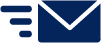# Manufacturing Charts

## Average Revenue Per Transaction

Explanation: Shows the revenue per individual transaction, as a monthly average.

Formula: Trading revenue / Sales - transactions

Report codes used: REV.TRA, SAL.TRA

## Debt Burden Percentage

Explanation: Measures the cost of servicing debt. This calculates the interest expense as a percentage of overall expenses, less cost of sales.

Formula: (Interest Expense / (Total Expenses - Cost of Sales)) * 100

Report codes used: EXP.INT, EXP, EXP.COS

## Direct Costs % to Revenue

Explanation: Shows direct wages costs and material costs as a percentage of trading revenue.

Formula:

• Direct Wages: Direct Wages / Trading Revenue * 100,
• Materials: (Cost of goods sold - Direct Wages) / Trading Revenue * 100

Report codes used: EXP.COS.WAG, EXP.COS, REV.TRA

## Labour Cost Per Unit

Explanation: Measures the cost of labour per unit of output.

Formula: Direct Wages / Volume

Report codes used: EXP.COS.WAG, OPR.VOL

## Materials Cost Per Unit

Explanation: Measures the cost of materials per unit of output.

Formula: (Cost of Goods Sold - Direct Wages) / Volume

Report codes used: EXP.COS, EXP.COS.WAG, OPR.VOL

## Stock Turn

Explanation: Shows the number of times stock is sold or used in a time period (per month). A low turnover may point to overstocking, obsolescence, or deficiencies. A high turnover may indicate inadequate inventory levels, which can lead to a loss in business.

Formula: Purchases / Average Closing Inventory

Report codes used: EXP.COS.PUR, ASS.CUR.INY

## Top Three Cost of Sales

Explanation: Shows the top three cost of sales accounts individually. Values for all other cost of sales accounts appear as 'Other'.

Formula: Cost of sales [YTD]

Report codes used: EXP.COS

## Units Manufactured

Explanation: Shows the total number of units manufactured, taken from the 'Volume' non-financial account.

Formula: Volume

Report codes used: OPR.VOL

## Units Per Transaction

Explanation: Measures the average number of items that customers are purchasing per transaction.

Formula: Items sold / sales transactions

Report codes used: SAL.ITE, SAL.TRAStill need help?
Get in touch!